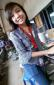# Solution to Problem 450 | Relationship Between Load, Shear, and Moment

3 posts / 0 new
Arra Clarize Ma...Solution to Problem 450 | Relationship Between Load, Shear, and Moment

Good day. May I ask if the 900 lb load in C is really downward? I think it is upward to maintain the shear of the 900 right? If it would be downward then the shear will be zero? Correct me if I'm wrong. Thanks!

Arra Clarize Ma...Jhun VertThe shear at C is 900 as indicated in the diagram

It is from 900 and goes to zero, that is why it is downward. If the load is upward, the shear diagram will not go to zero. It will be from 900 to 1800 instead.

• Mathematics inside the configured delimiters is rendered by MathJax. The default math delimiters are $$...$$ and $...$ for displayed mathematics, and $...$ and $...$ for in-line mathematics.